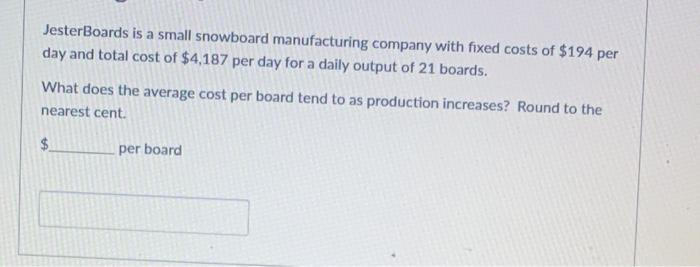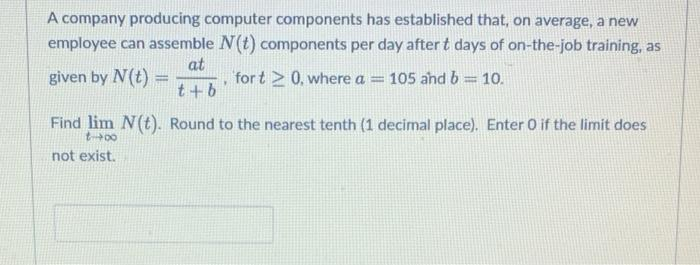Home / Expert Answers / Calculus / jesterboards-is-a-small-snowboard-manufacturing-company-with-fixed-costs-of-194-per-day-an-pa430

# (Solved): JesterBoards is a small snowboard manufacturing company with fixed costs of $$\ 194$$ per day an ...JesterBoards is a small snowboard manufacturing company with fixed costs of $$\ 194$$ per day and total cost of $$\ 4,187$$ per day for a daily output of 21 boards. What does the average cost per board tend to as production increases? Round to the nearest cent. per board A company producing computer components has established that, on average, a new employee can assemble $$N(t)$$ components per day after $$t$$ days of on-the-job training, as given by $$N(t)=\frac{a t}{t+b}$$, for $$t \geq 0$$, where $$a=105$$ and $$b=10$$. Find $$\lim _{t \rightarrow \infty} N(t)$$. Round to the nearest tenth (1 decimal place). Enter 0 if the limit does not exist.

We have an Answer from Expert